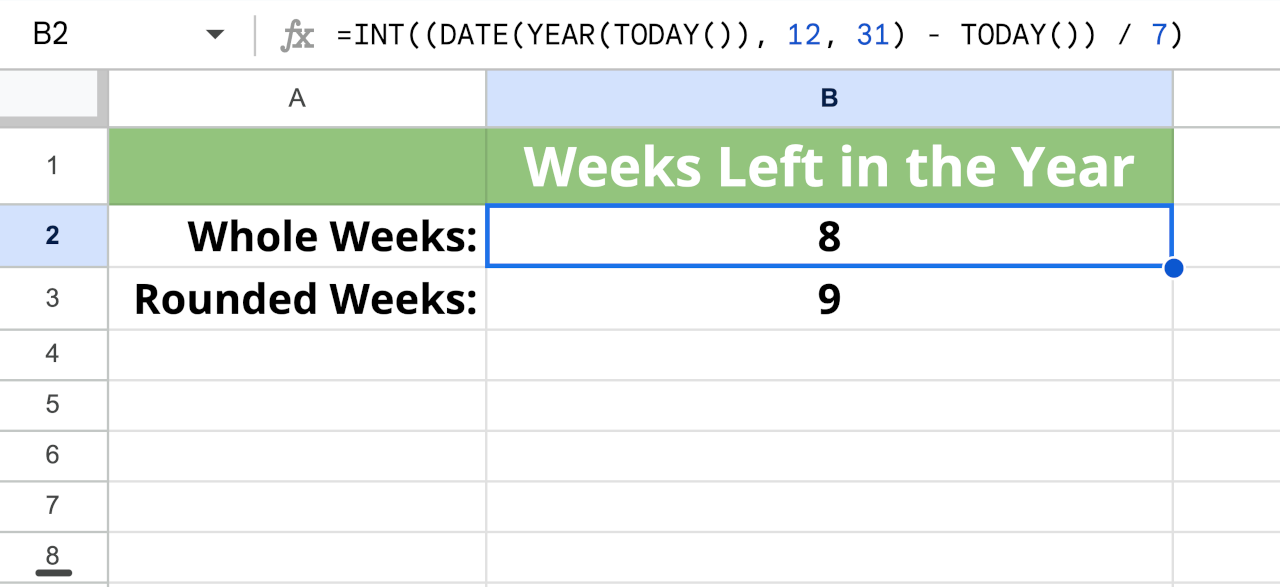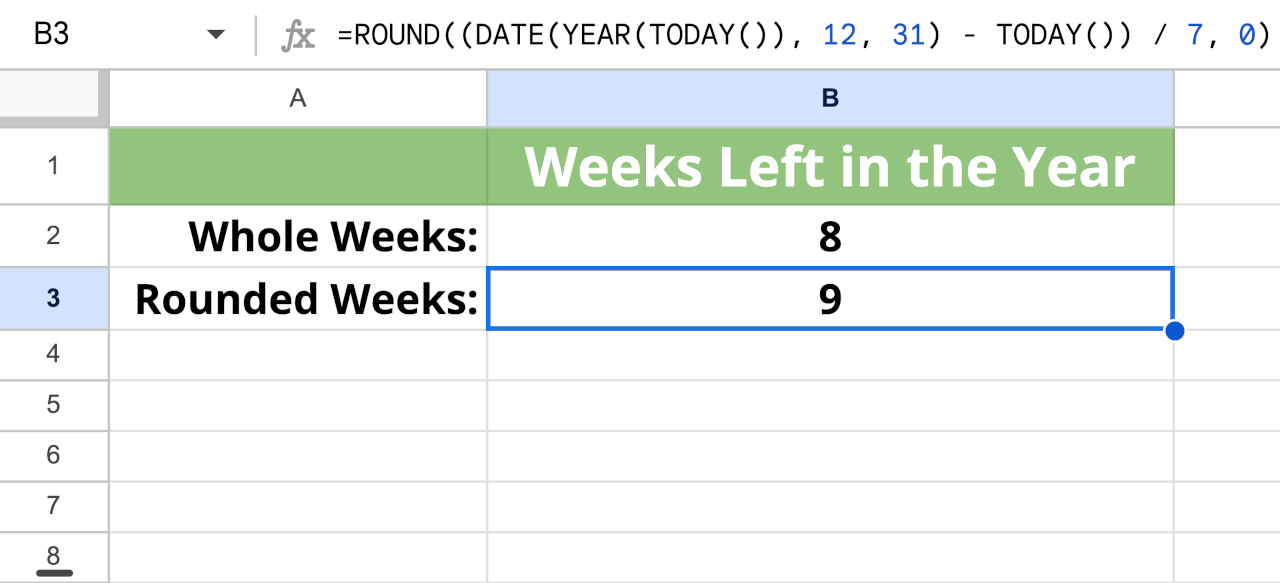# How Many Weeks Are Left in the Year? – Weeks Left in 2023

Are you trying to figure out how many weeks are left in the year?## Weeks Left in 2023:

4 weeks and 1 day

## Time Remaining in the Year:

• 0 months 29 days
• 4 weeks and 1 day
• 29 days
• 696 hours

There are four weeks and one day remaining in 2023 from today's date, which is December 2, 2023. You can use our days until New Year's calculator to confirm this.

The following chart shows the number of weeks remaining in the year from today and various other days.
Start DateWeeks Left in the Year
November 28, 20234 weeks and 5 days
November 29, 20234 weeks and 4 days
November 30, 20234 weeks and 3 days
December 1, 20234 weeks and 2 days
December 2, 20234 weeks and 1 day
December 3, 20234 weeks
December 4, 20233 weeks and 6 days
December 5, 20233 weeks and 5 days
December 6, 20233 weeks and 4 days

## How To Calculate How Many Weeks Are Left in the Year

There are a few methods to count the weeks left in 2023. The simplest is to use a calculator, such as our weeks between dates calculator. You can also calculate the weeks remaining this year manually.

### Method One: Use a Calendar

The first method to finding how many weeks are left in the year is to use a calendar.

#### Step One: Find the Start Date

Begin by identifying the start date - you'll need to find the month and day.

#### Step Two: Find the Days Remaining in the Start Month

Beginning with the start month, subtract the day in the start month from the total number of days in the month.

For instance, if the start date is June 3rd, since there are 30 total days in June, there are 30 - 3 = 27 days left in June.

#### Step Three: Find the Days in the Remaining Months

If there are any remaining full months in the year after the start month, then add the days in each month to the total days.

For instance, if the date range is from June 3 to December 31, then you'll need to add the days of July through December to the remaining days in June.

#### Step Four: Calculate the Total Days Left

The next step is to add up the days from steps two and three to calculate the total number of days left in the year.

#### Step Five: Calculate the Number of Weeks Left in the Year

The final step is to convert the number of days to weeks to find the weeks left in the year. To do this, divide the number of days left in the year by 7 to calculate the number of weeks left.

### Method Two: Subtract the Current Week Number

Another method you can use to count the weeks remaining in the year is to subtract the current week number from the total number of weeks in the year (either 52 or 53).

weeks left in 2023 = 52 – week number

To use this method, you need to know the current week of the year.

### Method Three: Use Sheets or Excel

You can also calculate the number of weeks remaining in 2023 in a spreadsheet such as Google Sheets or Microsoft Excel. You can do this with a few different formulas.

#### Whole Weeks Left in the Year

If you want to find the number of whole weeks left from the current day, you can use the following function:

=INT((DATE(YEAR(TODAY()), 12, 31) - TODAY()) / 7)

For instance, if the current date is October 31st, then the formula might look like this:#### Rounded Weeks Left in the Year

If you want to find the number of weeks left rounded to the closest whole week, you can use the following function:

=ROUND((DATE(YEAR(TODAY()), 12, 31) - TODAY()) / 7, 0)

For instance, if the current date is October 31st, then the formula might look like this:You can also use these formulas to calculate the date 4 weeks, 6 weeks, or even 8 weeks from now.